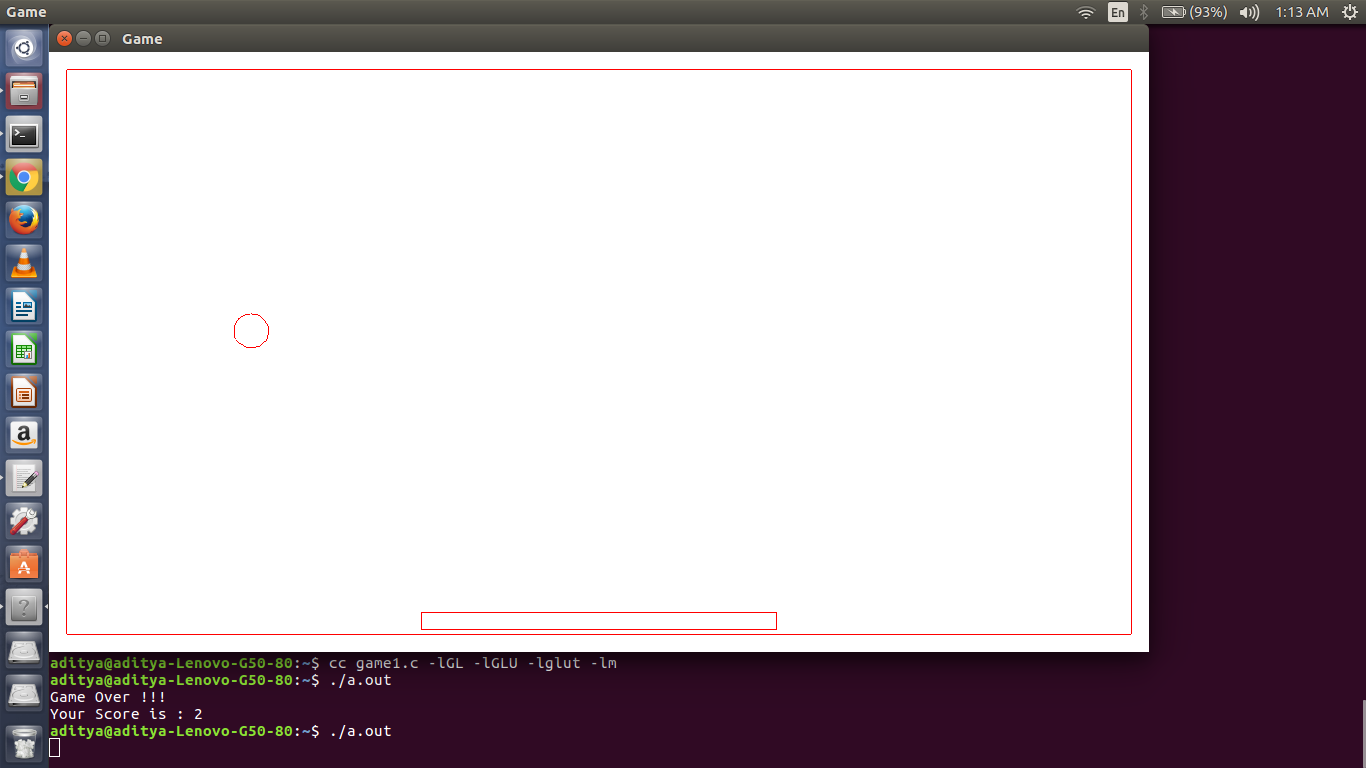OpenGL program for Simple Ball Game

• Difficulty Level : Expert
• Last Updated : 28 Dec, 2021

Prerequisite – OpenGL
OpenGL is a cross-language, cross-platform API for rendering 2D and 3D Vector Graphics. Using this, we can make a lot of design as well as animations. Below is a simple Game made using OpenGL.
Description : In this, a ball is moving starting from middle and goes to up-left in starting. But, then it collides with wall and changes direction but speed remain same. Consequently, it comes to downward direction where it should be catched on a rectangle (which is capable of moving in horizontal direction) in order to continue.
For working on Ubuntu operating system:

gcc filename.c -lGL -lGLU -lglut -lm
where filename.c is the name of the file
with which this program is saved.

C

 // C program to illustrate OpenGL game#include#include#include#define pi 3.142857 // Global Declaration// c and d tracks the number of time 'b' and 'n' pressed respectively// left and right indicates leftmost and rightmost index of movable rectangleint c = 0, d = 0, left = 0, right = 0;int m = 0, j = 1, flag1 = 0, l = 1, flag2 = 0, n = 0, score = 0, count = 1; // Initialization functionvoid myInit (void){    // Reset background color with white (since all three argument is 1.0)    glClearColor(1.0, 1.0, 1.0, 0.0);     // Set picture color to red (in RGB model)    // as only argument corresponding to R (Red) is 1.0 and rest are 0.0    glColor3f(1.0f, 0.0f, 0.0f);     // Set width of point to one unit    glPointSize(1.0);    glMatrixMode(GL_PROJECTION);    glLoadIdentity();     // Set window size in X- and Y- direction    gluOrtho2D(-620.0, 620.0, -340.0, 340.0);} // keyboard function : it gets active when button pressedvoid keyboard(unsigned char key, int x, int y){    left = -200 + 200 * (d - c);    right = 200 + 200 * (d - c);     // if leftmost index of window is hit    // then rectangle will not move to left on further pressing of b    // only it will move to right on pressing n    if (left == -600)    {        // '110' -> Ascii value of 'n'        // so d is incremented when n is pressed        if (key == 110)            d++;    }    // if rightmost index of window is hit    // then rectangle will not move to right on further pressing of n    // only it will move to left on pressing b    else if (right == 600)    {        // '98' -> Ascii value of 'b'        // so c is incremented when b is pressed        if (key == 98)            c++;    }    // when rectangle is in middle, then it will move into both    // direction depending upon pressed key    else    {        if (key == 98)            c++;           if (key == 110)            d++;    }    glutPostRedisplay();} void myDisplay(void){    // x and y keeps point on circumference of circle    int x, y, k;    // outer 'for loop' is to for making motion in ball    for (k = 0; k <= 400; k += 5)    {           glClear(GL_COLOR_BUFFER_BIT);        glBegin(GL_LINE_STRIP);        // i keeps track of angle        float i = 0;        // change in m denotes motion in vertical direction and        // change in n denotes motion in horizontal direction        m = m + 6;        n = n + 4;        // drawing of circle centre at (0, 12) iterated up to 2*pi, i.e., 360 degree        while (i <= 2 * pi)        {            y = 12 + 20 * cos(i);            x = 20 * sin(i);            i = i + 0.1;            // flag1 is 0 to show motion in upward direction and is 1 for downward direction            if (m == 288 && flag1 == 0)            {                j = -1;                m = -288;                flag1 = 1;                score++;            }            if (m == 288 && flag1 == 1)            {                j = 1;                m = -288;                flag1 = 0;            }            // flag2 is 0 to show motion in rightward direction and is 1 for leftward direction            if (n == 580 && flag2 == 0)            {                l = -1;                n = -580;                flag2 = 1;            }            if (n == 580 && flag2 == 1)            {                l = 1;                n = -580;                flag2 = 0;            }            // equation for desired motion of ball            glVertex2i((x - l * n), (y - j * m));        }        glEnd();         // these four points draws outer rectangle which determines window        glBegin(GL_LINE_LOOP);            glVertex2i(-600, -320);            glVertex2i(-600, 320);            glVertex2i(600, 320);            glVertex2i(600, -320);        glEnd();         // these four points draws smaller rectangle which is for catching ball        glBegin(GL_LINE_LOOP);        left = -200 + 200 * (d - c);        right = 200 + 200 * (d - c);            glVertex2i(left, -315);            glVertex2i(left, -295);            glVertex2i(right, -295);            glVertex2i(right, -315);        glEnd();         // following condition checks if falling ball is catched on rectangle or not        if ((j * m) == 276)        {            if ((left > ((-1 * l * n) + 20)) || (right < (-1 * l * n) - 20))            {                printf("Game Over !!!\nYour Score is :\t%d\n", score);                exit(0);            }        }        glutSwapBuffers();    }}  // Driver Programint main (int argc, char** argv){    glutInit(&argc, argv);         glutInitDisplayMode(GLUT_DOUBLE | GLUT_RGBA);    // Declares window size    glutInitWindowSize(1100, 600);         // Declares window position which is (0, 0)    // means lower left corner will indicate position (0, 0)    glutInitWindowPosition(0, 0);     // Name to window    glutCreateWindow("Game");     // keyboard function    glutKeyboardFunc(keyboard);    // Call to myInit()    myInit();    glutDisplayFunc(myDisplay);    glutMainLoop();}

Below is the screenshot of the animation. This screenshot is of one instance. In actual, ball will move in different direction which should be catched on the smaller rectangle. Rectangle can be moved to left by pressing lowercase b and towards right by lowercase n. However, this can be changed by changing the code in keyboard function where ASCII value of key can be changed.